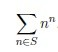favorite We need a little bit of your help to keep things running, click on this banner to learn more
Competitions

# Happy sum

It is known that the number is happy, if its decimal notation contains only fours and sevens. For example, the numbers 4, 7, 47, 7777 and 4744474 are happy.

Let S be the set of happy numbers, no less than a and no more than b: S = {n : anb, n is happy}. Calculate the remainder of dividing by 1234567891 the next sum:#### Input

Two integers a and b (1ab1018).

#### Output

Output the remainder of dividing the lucky sum by 1234567891.

#### Explanation

44 + 77 = 823799.

Time limit 1 second
Memory limit 128 MiB
Input example #1
1 10
Output example #1
823799
Source 2016 Summer Programming School, Uzhgorod, Day 1, Vasyl Biletskiy Contest